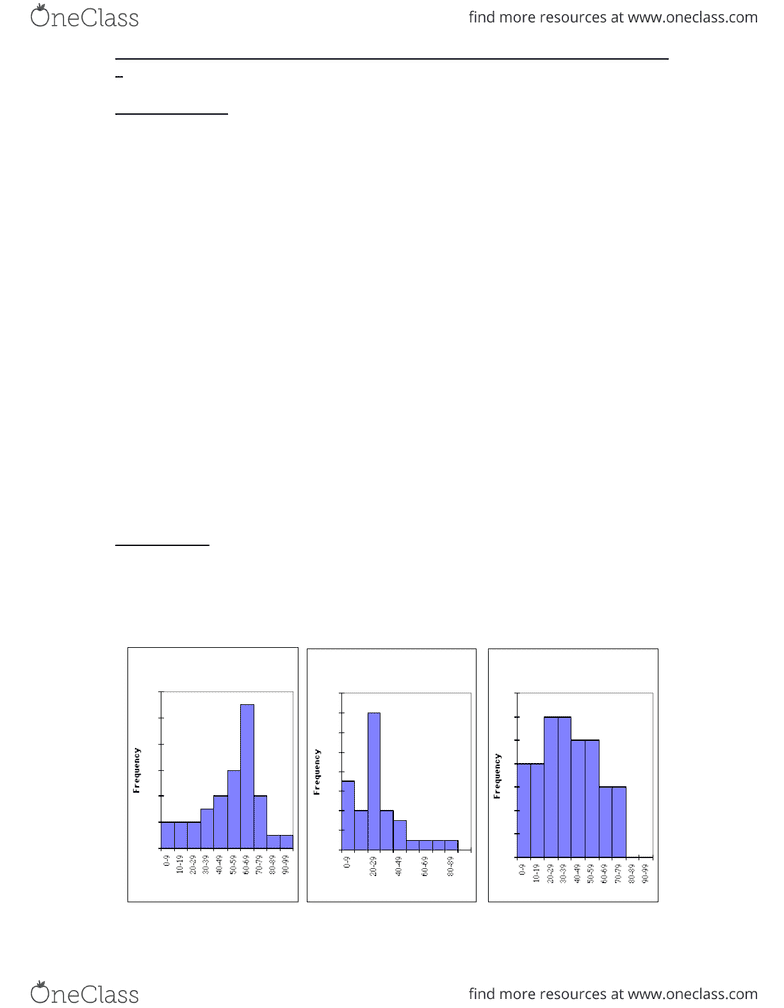Class Notes (1,100,000)
CA (620,000)
UW (20,000)
ENVS (200)
ENVS178 (30)
Lecture 4

# ENVS178 Lecture Notes - Lecture 4: Unimodality, Weighted Arithmetic Mean, Kurtosis

Department
Environmental Studies
Course Code
ENVS178
Professor
Jeff Casello
Lecture
4

This preview shows pages 1-3. to view the full 9 pages of the document.ENVS 178 LECTURE NOTE AIDS
DESCRIBING DISTRIBUTIONS: MEASURES OF SHAPE
Moments
The mean of a distribution is the balance point. A statistical synonym for mean or
average is the first moment.
The variance is based on the squared deviations from the mean and is called the second
moment (second because the deviations are squared).
The cubed deviations from the mean are used to calculate the third moment. Interestingly
enough the third moment tells us something about the shape and more particularly the
skewness.
Skewness
Skewed distributions are asymmetric about the mean. Skewed distributions have one tail
which is longer than the other and this causes an imbalance in the distribution.
Right-skewed:
»
Left-skewed:
»
There are several ways to estimate skewness.
One such measure is the 3rd moment, which is based on cubed differences.
The =skew function in Excel calculates skew in this way.
The formula is Σ[(xi – mean) / standard deviation]3 * n / [(n-1) (n-2)]
When xi < mean:
• the contribution of this xi value to the statistic is
»
• Because ‘differences’ are cubed, a few very small values of xi will result in a
summary number. A left-skew produces a negative summary number.
When xi > mean:
• the contribution of this xi value to the statistic is
»
• Because ‘differences’ are cubed, a few very large values of xi will result in a
summary number. A right-skew produces a positive summary number.

Only pages 1-3 are available for preview. Some parts have been intentionally blurred.ENVS 178 LECTURE NOTE AIDS
Cubed differences give very large numbers, however, and the unit of measurement is also
cubed. This makes it difficult to interpret these numbers.

Only pages 1-3 are available for preview. Some parts have been intentionally blurred.ENVS 178 LECTURE NOTE AIDS
A second measure is the Pearsonian skewness index or “coefficient of skewness”
3 (Mean – Median) / Standard Deviation
The sign of the Pearsonian skewness index value is based on the difference between the
mean and median. Does this make sense?
Why multiply by the constant “3”?
»
What happens when this difference is divided by the standard deviation?
»
What would be the direction (and sign) of the skew in each of the following cases?
• When the mean equals the median
»
• When the mean is less than the median
»
• When the mean is greater than the median
»
A third method is to visually inspect a graphic display of the data.
This example shows the age distribution of a sample of residents from each of three
communities. Indicate the mean and median on each of the graphs. What is the direction
and magnitude of the skew in each case? What would explain these differences?
Age of Residents of Retirement
Community
0
2
4
6
8
10
12
Age of Residents of Resource
Town
0
2
4
6
8
10
12
14
16
Age of Residents of Service
Centre
0
1
2
3
4
5
6
7
###### You're Reading a Preview

Unlock to view full version